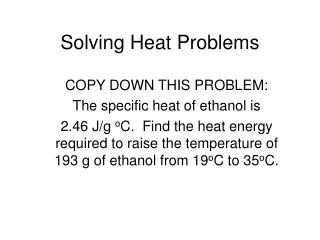DownloadDownload PresentationSolving Heat Problems

# Solving Heat Problems

Télécharger la présentation## Solving Heat Problems

- - - - - - - - - - - - - - - - - - - - - - - - - - - E N D - - - - - - - - - - - - - - - - - - - - - - - - - - -
##### Presentation Transcript

1. Solving Heat Problems COPY DOWN THIS PROBLEM: The specific heat of ethanol is 2.46 J/g oC. Find the heat energy required to raise the temperature of 193 g of ethanol from 19oC to 35oC.

2. Step 1 • What type of problem is it? Heat • What is the question? Find the heat energy • What equation to use? Q = mCpDT • Does the equation need to be manipulated? No manipulation needed

3. Step 2 • Plug in the numbers • m = 193 g • Cp = 2.46 J/g-oC • DT = 35 – 19 = 16 oC • Soooo, Q = 193g x 2.46 J/g-oC x 16 oC

4. Step 3 • Calculate Answer is 7596.48 • Cancel Units (if you don’t end up with the right units, you haven’t used appropriate equation!) All cancel except J, which is the unit for energy • Determine Significant Figures There are 2 significant figures in the temp., so two should be in the answer. • Write your final answer. 7600 J or 7.6 x 103 J

5. Problem 2 • When a 120 g sample of aluminum absorbs 9612 J of heat energy, its temperature increases from 25oC to 115oC. Find the specific heat of aluminum. Include the correct unit. • Type of Problem? • Question? • Equation? Does it Need to Be Manipulated?

6. Equation Manipulation • Q = mCpDT, but you need Cp, so divide both sides by m and DT. So the final equation is Cp = Q m DT You may notice the equation mirrors the units for Cp of J/g-oC.

7. Plug in the Numbers Cp = Q/mDT so, Cp = 9612 J/(120g x 90 oC) The mathematical answer is 0.89, but there is only one sig. fig. in 90, so the answer is 0.9 J/g-oC

8. Check for Understanding • Complete conversion problems #1-3 to see if you are able to convert from one heat unit to another. • Then, complete heat problems #1 and #4 on the worksheet to check your understanding of working that type of problem. Work more of these for practice at home (answers will be on the blog for you to self check). Quiz Monday!

9. A Challenge Problem • The specific heat of lead is 0.129 J/goC. Find the amount of heat released when 2.4 mol of lead are cooled from 37.2oC to 22.5oC. WATCH THE UNITS!!